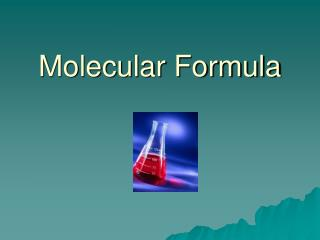# Molecular Formula - PowerPoint PPT PresentationDownload PresentationMolecular Formula

Molecular FormulaDownload Presentation## Molecular Formula

- - - - - - - - - - - - - - - - - - - - - - - - - - - E N D - - - - - - - - - - - - - - - - - - - - - - - - - - -
##### Presentation Transcript

1. Molecular Formula

2. Chapter 7.7Molecular Formula Objective: To calculate the molecular formula for a compound.

3. Determining a Molecular Formula from an Empirical Formula Step 1: Find the molar mass of the empirical formula. Step 2: Divide the molar mass of the molecular formula by the molar mass of the empirical formula. Step 3: Multiply the empirical formula by the factor obtained in step 2.

4. Example #1 The empirical formula for a compound is P2O5. Its experimental molar mass is 284 g/mol. Determine the molecular formula of the compound.

5. Example #1 The empirical formula for a compound is P2O5. Its experimental molar mass is 284 g/mol. Determine the molecular formula of the compound. Step 1: The molar mass of P2O5 is 141.94 g/mol Step 2: (284 g/mol) / (141.94 g/mol) = 2.00 Step 3: 2 x (P2O5) = P4O10

6. Example #2 Determine the molecular formula for a compound that has a molar mass of 232.41 g/mol and an empirical formula of OCNCl.

7. Example #2 Determine the molecular formula for a compound that has a molar mass of 232.41 g/mol and an empirical formula of OCNCl. Step 1:The molar mass of OCNCl is 77.47 g/mol Step 2: (232.41 g/mol) / (77.47 g/mol) = 3.00 Step 3: 3 x (OCNCl) = O3C3N3Cl3

8. Example #3 Determine the molecular formula for a compound that has a molar mass of 32.06 g/mol and an empirical formula of NH2.

9. Example #3 Determine the molecular formula for a compound that has a molar mass of 32.06 g/mol and an empirical formula of NH2. Step 1:The molar mass of NH2 is 16.01 g/mol Step 2: (32.06 g/mol) / (16.01 g/mol) = 2.00 Step 3: 2 x (NH2) = N2H4

10. Example #4 Determine the molecular formula for a compound that has a molar mass of 120.12 g/mol and an empirical formula of CH2O.

11. Example #4 Determine the molecular formula for a compound that has a molar mass of 120.12 g/mol and an empirical formula of CH2O. Step 1:The molar mass of CH2O is 30.01 g/mol Step 2: (120.12 g/mol) / (30.01 g/mol) = 4.00 Step 3: 4 x (CH2O) = C4H8O4